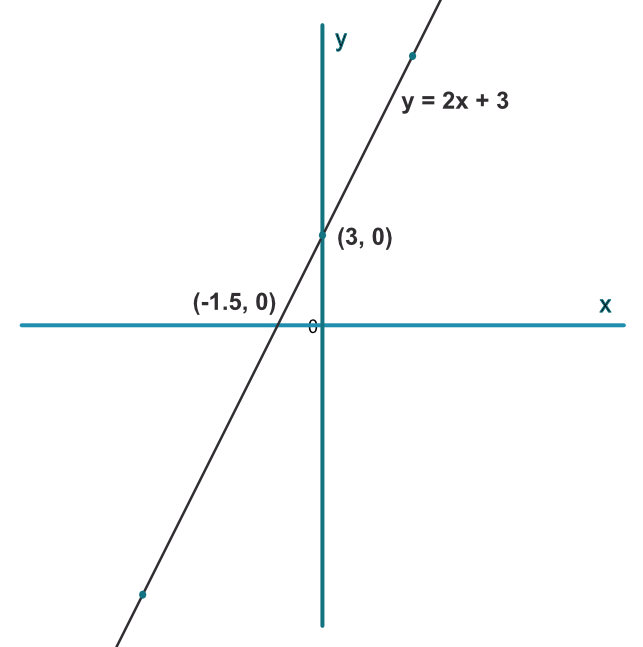Plotting Graphs

## Plotting Graphs

Before plotting a graph, make sure that the equation has a single y on one side of the equals sign, and the x values are all on the other side. If the graph to be plotted is given as a function (f(x)), then it has already been correctly arranged.

Plot the graph as instructed in the question. For linear graphs, use a straight edge. For other graphs, draw a clear line keeping curves as regular as possible and passing through all the points.

If a sketch has been requested, show on the sketch the coordinates for the intercept with the y-axis, the roots of the equation (crossing the x-axis) and any turning points. A ruler must be used for the sketch - for the axis and any linear function.

## Example 1

Sketch the graph for y=2x+3. Show the coordinates of the intercept points on the x-axis and the y-axis.

 x -6 0 3 2x+3

Complete the table as indicated. Solve the equation for y=2x+3 for the intercept on the x-axis.

2(-6) + 3 = -9

2(0) = 3 = 3

2(3) + 3 = 9

 x -6 0 3 2x+3 -9 3 9## Example 2

Complete the following table for the equation 4x^2=y + 6x - 2

 x -5 -3 -1 1 3 5 ? 132 ? 12 ? 20 72

Rearrange the equation y+6x-2=4x^2

y+6x=4x^2+2

y=4x^2-6x+2

And substitute for the two missing values

y=4(-3)^2-6(-3)+2=56

y=4(1)^2-6(-1)+2=0

 x -5 -3 -1 1 3 5 4x^2-6x+2 132 56 12 0 20 72